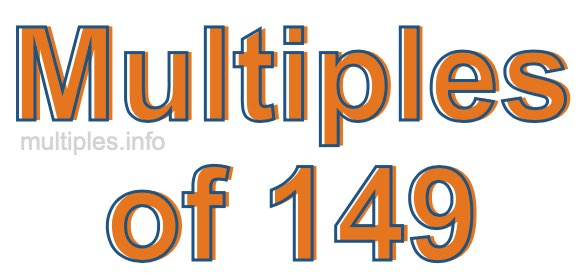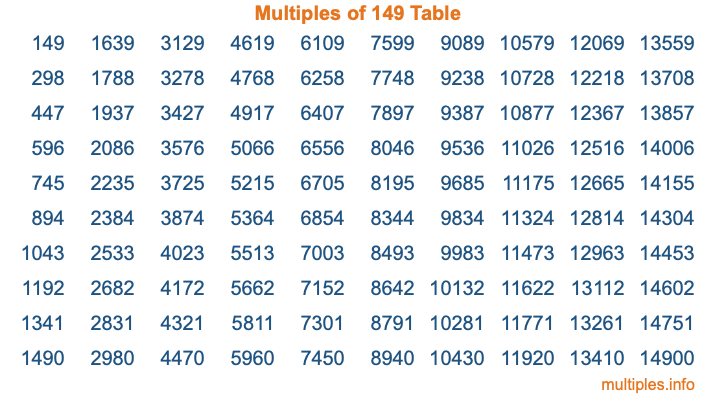Multiples of 149Welcome to the Multiples of 149 page. Here we will first teach you everything you will ever need to know about the multiples of 149, and then give you a study guide summary of everything we taught you to make sure you remember it all. Use this page to look up facts and learn information about the multiples of 149. This page will make you a multiples of one hundred forty-nine expert!

Definition of Multiples of 149
Multiples of 149 are all the numbers that when divided by 149 equal an integer. Each of the multiples of 149 are called a multiple. A multiple of 149 is created by multiplying 149 by an integer.

Therefore, to create a list of multiples of 149, you start with 1 multiplied by 149, then 2 multiplied by 149, then 3 multiplied by 149, and so on for as long as you want. Thus, the list of the first five multiples of 149 is 149, 298, 447, 596, and 745. To see a larger list of multiples of 149, see the printable image of Multiples of 149 further down on this page. We also have a category where you can choose any nth multiple of 149.

Multiples of 149 Checker
The Multiples of 149 Checker below checks to see if any number of your choice is a multiple of 149. In other words, it checks to see if there is any number (integer) that when multiplied by 149 will equal your number. To do that, we divide your number by 149. If the the quotient is an integer, then your number is a multiple of 149.

Is  a multiple of 149?

Least Common Multiple of 149 and ...
A Least Common Multiple (LCM) is the lowest multiple that two or more numbers have in common. This is also called the smallest common multiple or lowest common multiple and is useful to know when you are adding our subtracting fractions. Enter one or more numbers below (149 is already entered) to find the LCM.

Check out our LCM Calculator if you need more details about the Least Common Multiple or if you need the LCM for different numbers for adding and subtraction fractions.

nth Multiple of 149
As we stated above, 149 is the first multiple of 149, 298 is the second multiple of 149, 447 is the third multiple of 149, and so on. Enter a number below to find the nth multiple of 149.

th multiple of 149

Multiples of 149 vs Factors of 149
149 is a multiple of 149 and a factor of 149, but that is where the similarities end. All postive multiples of 149 are 149 or greater than 149. All positive factors of 149 are 149 or less than 149.

Below is the beginning list of multiples of 149 and the factors of 149 so you can compare:

Multiples of 149: 149, 298, 447, 596, 745, etc.

Factors of 149: 1, 149

As you can see, the multiples of 149 are all the numbers that you can divide by 149 to get a whole number. The factors of 149, on the other hand, are all the whole numbers that you can multiply by another whole number to get 149.

It's also interesting to note that if a number (x) is a factor of 149, then 149 will also be a multiple of that number (x).

Multiples of 149 vs Divisors of 149
The divisors of 149 are all the integers that 149 can be divided by evenly. Below is a list of the divisors of 149.

Divisors of 149: 1, 149

The interesting thing to note here is that if you take any multiple of 149 and divide it by a divisor of 149, you will see that the quotient is an integer.

Multiples of 149 Table
Below is an image of the first 100 multiples of 149 in a table. The table is in chronological order, column by column. The first column has the first ten multiples of 149, the second column has the next ten multiples of 149, and so on.The Multiples of 149 Table is also referred to as the 149 Times Table or Times Table of 149. You are welcome to print out our table for your studies.

Negative Multiples of 149
Although not often discussed or needed in math, it is worth mentioning that you can make a list of negative multiples of 149 by multiplying 149 by -1, then by -2, then by -3, and so on, to get the following list of negative multiples of 149:

-149, -298, -447, -596, -745, etc.

Multiples of 149 Summary
Below is a summary of important Multiples of 149 facts that we have discussed on this page. To retain the knowledge on this page, we recommend that you read through the summary and explain to yourself or a study partner why they hold true.

There are an infinite number of multiples of 149.

A multiple of 149 divided by 149 will equal a whole number.

149 divided by a factor of 149 equals a divisor of 149.

The nth multiple of 149 is n times 149.

The largest factor of 149 is equal to the first positive multiple of 149.

149 is a multiple of every factor of 149.

149 is a multiple of 149.

A multiple of 149 divided by a divisor of 149 equals an integer.

149 divided by a divisor of 149 equals a factor of 149.

Any integer times 149 will equal a multiple of 149.

Multiples of a Number
Here you can get the multiples of another number, all with the same attention to detail as we did for multiples of 149 on this page.

Multiples of
Multiples of 150
Did you find our page about multiples of one hundred forty-nine educational? Do you want more knowledge? Check out the multiples of the next number on our list!